# The Law of Iterated Expectation Nancy Zhang Easy

• Slides: 9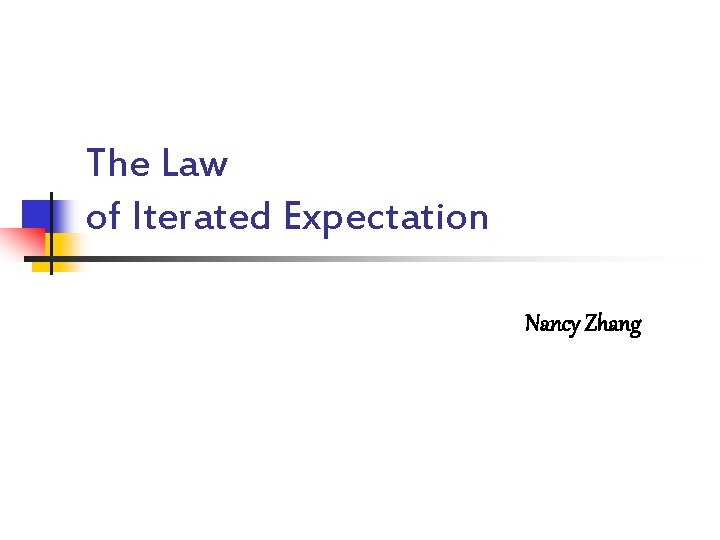The Law of Iterated Expectation Nancy Zhang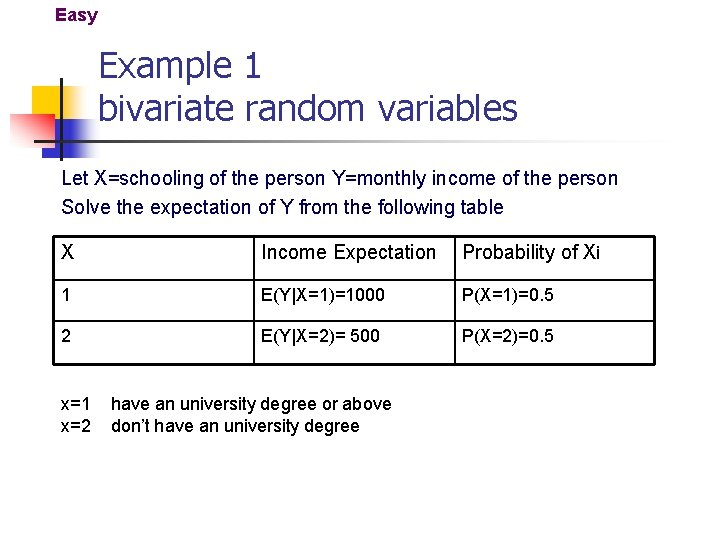Easy Example 1 bivariate random variables Let X=schooling of the person Y=monthly income of the person Solve the expectation of Y from the following table X Income Expectation Probability of Xi 1 E(Y|X=1)=1000 P(X=1)=0. 5 2 E(Y|X=2)= 500 P(X=2)=0. 5 x=1 x=2 have an university degree or above don’t have an university degree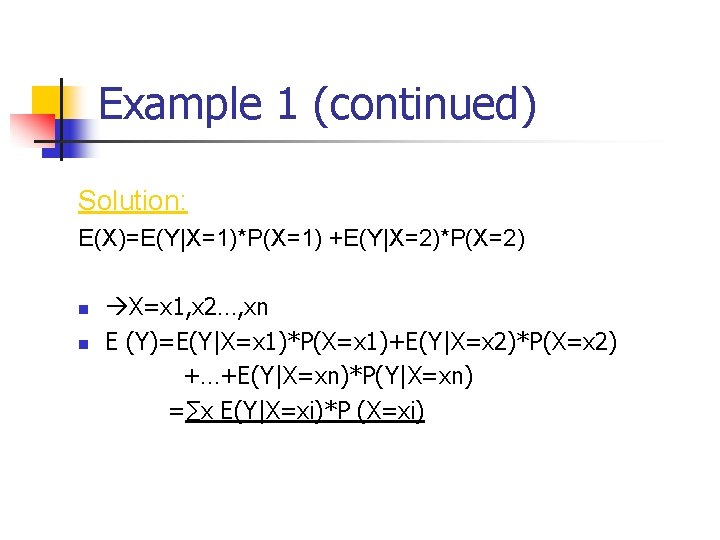Example 1 (continued) Solution: E(X)=E(Y|X=1)*P(X=1) +E(Y|X=2)*P(X=2) n n X=x 1, x 2…, xn E (Y)=E(Y|X=x 1)*P(X=x 1)+E(Y|X=x 2)*P(X=x 2) +…+E(Y|X=xn)*P(Y|X=xn) =∑x E(Y|X=xi)*P (X=xi)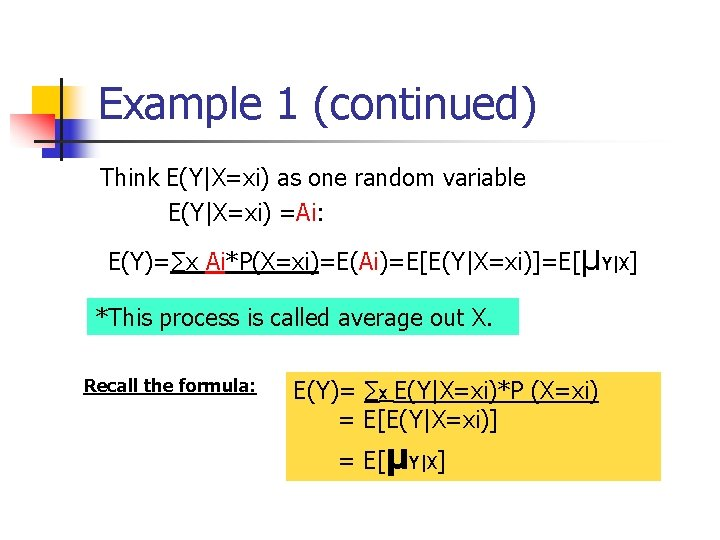Example 1 (continued) Think E(Y|X=xi) as one random variable E(Y|X=xi) =Ai: E(Y)=∑x Ai*P(X=xi)=E(Ai)=E[E(Y|X=xi)]=E[μY|X] *This process is called average out X. Recall the formula: E(Y)= ∑x E(Y|X=xi)*P (X=xi) = E[E(Y|X=xi)] = E[μY|X]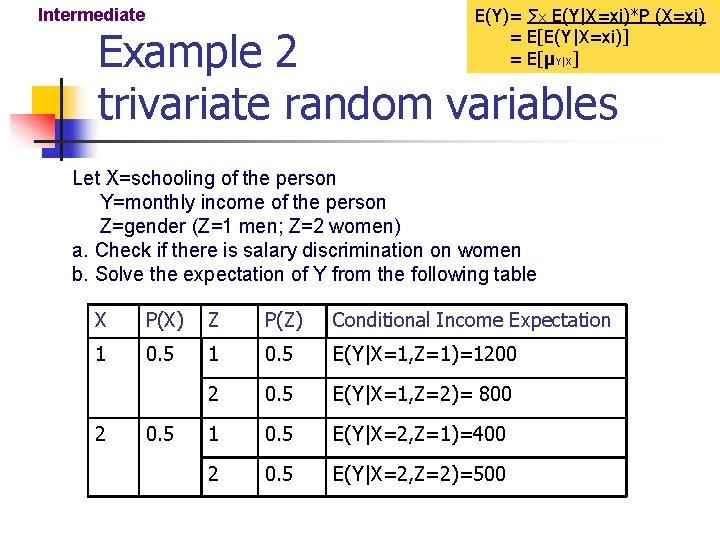E(Y)= ∑x E(Y|X=xi)*P (X=xi) = E[E(Y|X=xi)] = E[μY|X] Intermediate Example 2 trivariate random variables Let X=schooling of the person Y=monthly income of the person Z=gender (Z=1 men; Z=2 women) a. Check if there is salary discrimination on women b. Solve the expectation of Y from the following table X P(X) Z P(Z) Conditional Income Expectation 1 0. 5 E(Y|X=1, Z=1)=1200 2 0. 5 E(Y|X=1, Z=2)= 800 1 0. 5 E(Y|X=2, Z=1)=400 2 0. 5 E(Y|X=2, Z=2)=500 2 0. 5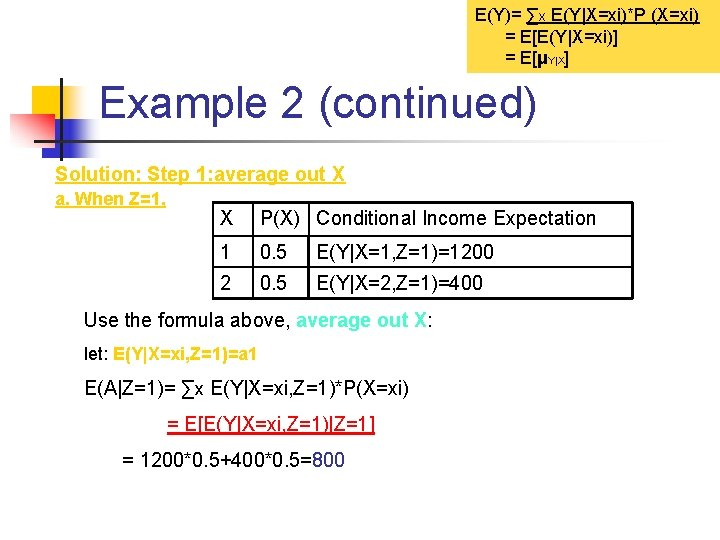E(Y)= ∑x E(Y|X=xi)*P (X=xi) = E[E(Y|X=xi)] = E[μY|X] Example 2 (continued) Solution: Step 1: average out X a. When Z=1, X P(X) Conditional Income Expectation 1 0. 5 E(Y|X=1, Z=1)=1200 2 0. 5 E(Y|X=2, Z=1)=400 Use the formula above, average out X: let: E(Y|X=xi, Z=1)=a 1 E(A|Z=1)= ∑x E(Y|X=xi, Z=1)*P(X=xi) = E[E(Y|X=xi, Z=1)|Z=1] = 1200*0. 5+400*0. 5=800E(Y)= ∑x E(Y|X=xi)*P (X=xi) = E[E(Y|X=xi)] = E[μY|X] Example 2 (continued) Similarly, X P(X) Conditional Income Expectation when Z=2, 1 0. 5 E(Y|X=1, Z=2)=800 2 0. 5 E(Y|X=2, Z=2)=500 average out X: E(A|Z=2) = ∑x E(Y|X=xi, Z=2)*P(X=xi) = 800*0. 5+500*0. 5=650 As 650<800, the conditional expectations suggest that there is salary discrimination on women. * *Note: self-created data, not based on real survey.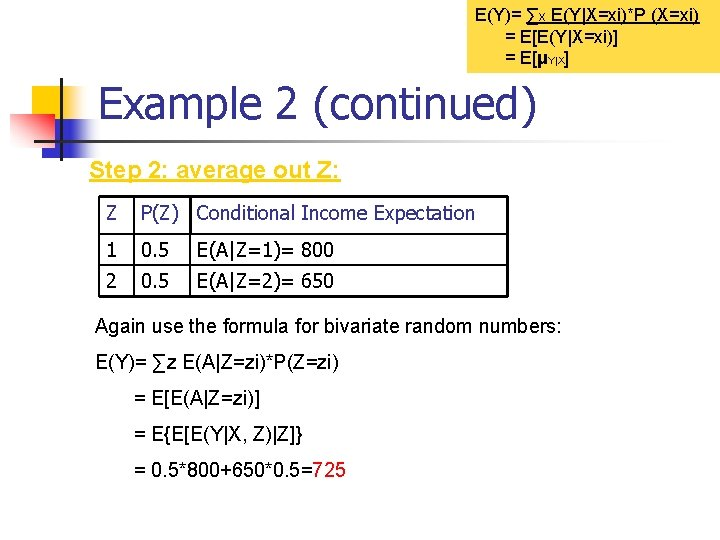E(Y)= ∑x E(Y|X=xi)*P (X=xi) = E[E(Y|X=xi)] = E[μY|X] Example 2 (continued) Step 2: average out Z: Z P(Z) Conditional Income Expectation 1 0. 5 E(A|Z=1)= 800 2 0. 5 E(A|Z=2)= 650 Again use the formula for bivariate random numbers: E(Y)= ∑z E(A|Z=zi)*P(Z=zi) = E[E(A|Z=zi)] = E{E[E(Y|X, Z)|Z]} = 0. 5*800+650*0. 5=725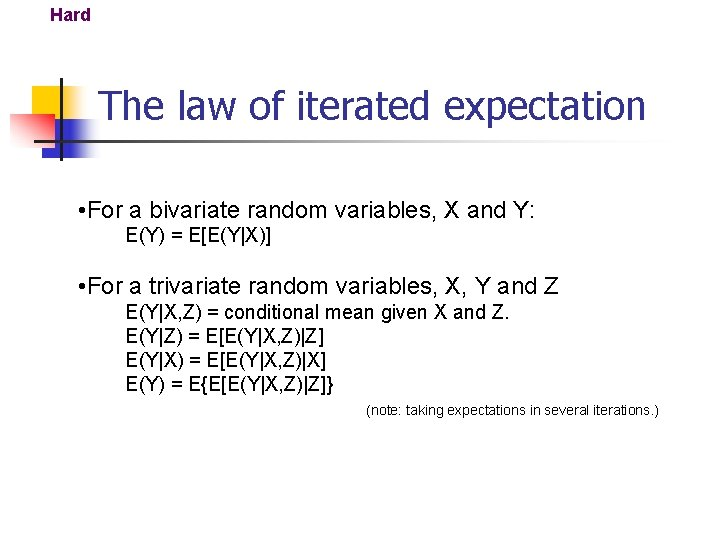Hard The law of iterated expectation • For a bivariate random variables, X and Y: E(Y) = E[E(Y|X)] • For a trivariate random variables, X, Y and Z E(Y|X, Z) = conditional mean given X and Z. E(Y|Z) = E[E(Y|X, Z)|Z] E(Y|X) = E[E(Y|X, Z)|X] E(Y) = E{E[E(Y|X, Z)|Z]} (note: taking expectations in several iterations. )